Courses

# Hydrostatics Force Civil Engineering (CE) Notes | EduRev

## Civil Engineering (CE) : Hydrostatics Force Civil Engineering (CE) Notes | EduRev

The document Hydrostatics Force Civil Engineering (CE) Notes | EduRev is a part of the Civil Engineering (CE) Course Civil Engineering SSC JE (Technical).
All you need of Civil Engineering (CE) at this link: Civil Engineering (CE)

Hydrostatics Force
Pressure Force on Plane Surfaces:

• Pressure force always acts normal to plane surface.
• The center of pressure passes through centroid of the pressure diagram.
• In horizontal plane surface pressure force passes through centroid of area whereas in inclined and vertical plane surfaces pressure force passes below the centroid of the area.
• The total pressure force on plane area is F = Area × Pressure at the centroid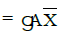where,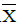= vertical depth of centroid of area from free liquid surface
A = Area of surface

• Depth of center of pressure from free surface is given by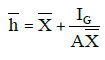where, IG = Moment of inertia about centre of gravity of the body.

• Center of pressure always lies below the centroid of the area in non-horizontal surfaces.
• The depth of center of pressure on inclined plane is given by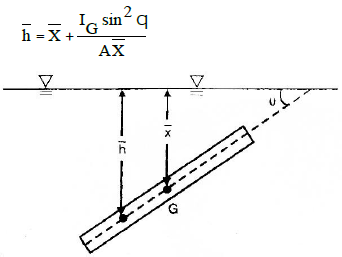-Pressure Force on curved surfaces: Horizontal component of the resultant hydrostatic force 'Fx' of curved surface may be computed by projecting the surface upon a vertical plane and multiplying the projected area by the pressure at its own centre of area.
-Vertical component of force 'Fy' is equal to the weight of the liquid block lying above the curved surface upto free surface.
Resultant Force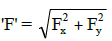-Angle of line of action of resultant force with the horizontal is given by tan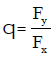-Depth of center of pressure for some vertical plane surfaces

 S.No Surface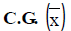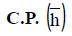1. RECTANGLE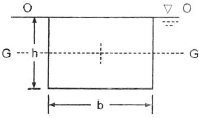(h/2) (2h/3) 2. TRAPEZIUM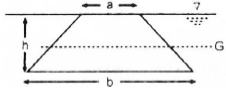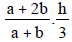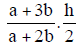3. TRIANGLE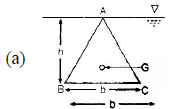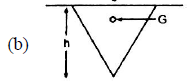(2h/3) (3h/4) 4. CIRCLE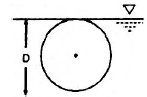(d/2) (5d/8) 5. SEMI CIRCLE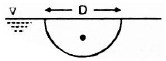2D/3π 3πD/32 6. PARABOLA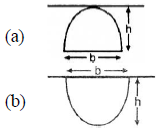(3h/5)        (2h/5) (5h/7)      (4h/7)

Archimedes principle and Force of buoyancy:

• When a body is submerged either fully or partially then it is acted upon by a force of buoyancy vertically up ward which is equal to weight of liquid displaced by the body. This force of buoyancy always acts through the centroid of liquid displaced.
• Stability of submerged bodies:If centre of buoyancy (B) and centre of gravity (G), coincide then submerged body is in neutral equilibrium & if B is above G it will be in stable equilibrium. If B is below G it will be in unstable equilibrium.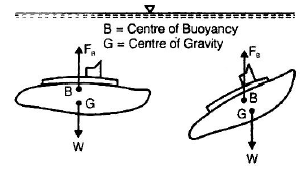Stability of floating bodies: The stability of floating body is defined by relative position of metacentre (M) and centre of gravity (G).
-A floating body is STABLE if, when it is displaced, it returns to equilibrium.
-A floating body is UNSTABLE if, when it is displaced, it moves to a new equilibrium.

-If M lies above G (GM = +ve) then it is stable equilibrium
-If M lies below G (GM = - ve) then unstable equilibrium
-If M coincides with G (GM = 0) then neutral equilibrium

GM =  (I/V) -(BG))
where GM = metacentric height.

I = moment of inertia about longitudinal axis of the plane at the level of free surface. ('I' is minimum moment of inertial of the plane.)
V = Volume of liquid displaced by the body.
BG = Distance between centroid of liquid displaced and centroid of the body.

For stable equilibrium of a circular cone floating with its apex downward having diameter 'd' and a vertical height 'h'. (The specific gravity of the cone is 'S'), The condition for stability is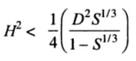Vortex Motion : A whirling mass of fluid is called vortex flow.

(i) Forced Vortex Flow:
When a fluid is rotated about a vertical axis at constant speed, such every particle of its has the same angular velocity, motion is known as the forced vortex.Forced vortex requires constant supply of external energy/torque.
h=ω2r2/2g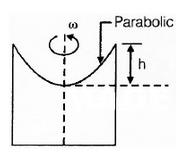where h is height of paraboloid, and r is radius of paraboloid at top.
Volume of paraboloid =  1/2 πr2h

The surface profile of forced vortex flow is parabolic.
Example of forced vortex flow is rotating cylinder & flow inside centrifugal pump.

(ii) Free Vortex Flow:
-In this flow fluid mass rotates due to conservation of angular momentum. No external torque or energy is required. In free vortex flow Bernoulli's equation can be applied. The velocity profile is inversely proportional with the radius.
v r = constant
-The point at the centre of rotation is called singular point, where velocity approaches to infinite.
-Example of free vortex motion are whirling mass of liquid in wash basin, whirlpool in rivers etc.

Offer running on EduRev: Apply code STAYHOME200 to get INR 200 off on our premium plan EduRev Infinity!

,

,

,

,

,

,

,

,

,

,

,

,

,

,

,

,

,

,

,

,

,

;